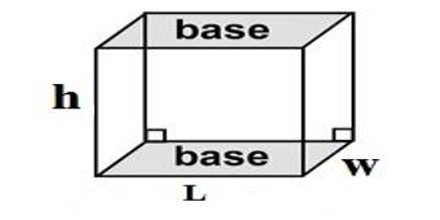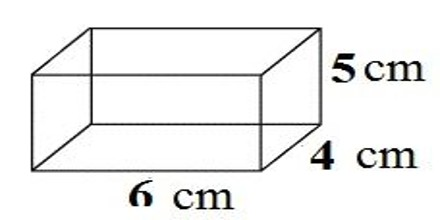Volume of a Rectangular Prism

Volume is the amount of 3-dimensional space an object occupies. Volume is measured in cubic units. To find the volume of a rectangular prism, we multiply the length of the prism by the width of the prism by the height of the prism.Explanation: Imagine a fish aquarium. Its length, width, and height determine how much water the tank will hold. If you fill it with water, the amount of water is the volume that the tank will hold.  You measure volume in cubic units, because you are multiplying three dimensions: length, width, and height.

The volume of a rectangular prism can be found by the formula:

volume = length x width x heightExample: find the volume of the Rectangular Prism

Formulas; is v = L * w * h

l = 6 cm

w = 4 cm

h = 5 cm

v = 6 * 4 * 5 = 120 cm³.